### Power System Analysis

Data is displayed for academic year: 2023./2024.

#### Course Description

Introduction to Power Systems Analysis. Network Equations. Network models: generators, lines and transformers, voltage control devices. Admittance and impedance matrices. Numerical methods for load flow. Gauss and Gauss-Seidel methods. Newton-Raphson and Fast Decoupled Load Flow. Sparse matrix algebra in network calculations. Simplified load flow models. DC models. 3-phase load flow. Fault analysis.

#### Study Programmes

[FER3-EN] Electrical Power Engineering - profile
(1. semester)

#### Learning Outcomes

1. analyse and model network components
2. create a network model for power flow calculations and fault analysis
3. identify and determine network matrices, as well as values of admittance and impedance matrices
4. apply numerical iterative methods for power flow calculations
5. calculate the state vector of an electric power system
6. select appropriate power flow methods depending on network conditions
7. calculate fault currents for different short-circuit types
8. select appropriate short-circuit calculation methods based on fault type and network conditions
9. analyse electric power system state and parameters

#### Forms of Teaching

Lectures

Lectures take place in a lecture room with a lecturer who is an expert on a specific subject. The lecture is interactive, and students are expected to participate in the discussion.

Seminars and workshops

Students work on two projects during the semester.

Exercises

Recitations include solving practical tasks and discussing solving procedures.

Laboratory

Laboratory exercises are held in labs equipped with computers. During the exercise, students try out theoretical concepts and apply them to specific problems.

Continuous Assessment Exam
Laboratory Exercises 0 % 10 % 0 % 10 %
Homeworks 0 % 10 % 0 % 10 %
Mid Term Exam: Written 8 % 25 % 0 %
Final Exam: Written 11 % 35 %
Final Exam: Oral 20 %
Exam: Written 25 % 50 %
Exam: Oral 30 %

#### Week by Week Schedule

1. Superposition, compensation, Thevening, Norton, Millman, node elimination, reciprocity
2. Simetrical components, The Per-Unit quantities
3. Network model of transmission lines, Network models of transformers with nominal and off-nominal ratios
4. Network models of phase-shifting transformers, Load and generator models
5. Incidence (connection) matrix, Network topology representation and modelling, Y matrix, Z matrix, Scarce matrix algebra for power systems calculations
6. Node clasification, Mathematical formulation of power flow
7. Gauss-Seidel with Y matix, Gauss-Seidel with Z matix
8. Midterm exam
9. Newton-Raphson method, Advanced power flow calculation based on accelerated Newton-Raphson method
10. Simplified power flow (DC model of AC grid), DC system model, Power flow on HVDC network, Power flow in low woltage grid
11. Short circuit currents importance, Clasical method (reduced impedance and per unit method), Matrix method, 3 phase short circuit
12. 2 phase and 2 phase with ground short circuit, 1 phase short circuit, Peak current, thermal current, breaking current
13. On-line and off line fault analysis, Limitation of short circuit currents, Influence of neutral earthing
15. Final exam

#### Literature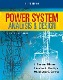J. Duncan Glover, Thomas Overbye, Mulukutla S. Sarma (2016.), Power System Analysis and Design, Cengage Learning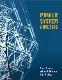John J. Grainger, William D. Stevenson, Gary W. Chang (2016.), Power System Analysis, McGraw-Hill Education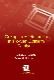Glenn W. Stagg, Ahmed H. El-Abiad (2019.), Computer Methods in Power System Analysis, Medtech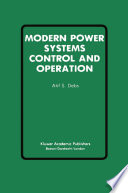Atif S. Debs (2012.), Modern Power Systems Control and Operation, Springer Science & Business Media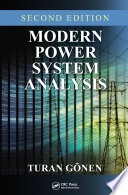Turan Gonen (2016.), Modern Power System Analysis, CRC Press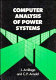Jos Arrillaga, C. P. Arnold (1990.), Computer Analysis of Power Systems, John Wiley & Sons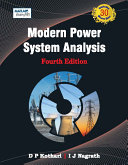D. P. Kothari, I. J. Nagrath (2011.), Modern Power System Analysis, Tata McGraw-Hill Education

#### General

ID 222919
Winter semester
5 ECTS
L1 English Level
L1 e-Learning
45 Lectures
5 Seminar
15 Exercises
8 Laboratory exercises
0 Project laboratory